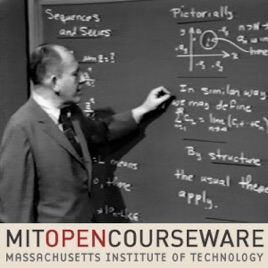20 episodes

This course gives an introduction to Complex Variables, Ordinary Differential Equations and Linear Algebra.# Calculus Revisited: Calculus of Complex Variables, Differential Equations, and Linear Algebra MIT

• Education

This course gives an introduction to Complex Variables, Ordinary Differential Equations and Linear Algebra.

• video
Part I: Complex Variables, Lecture 1: The Complex Numbers

## Part I: Complex Variables, Lecture 1: The Complex Numbers

Herb Gross explains the need to define complex numbers. He defines the structure of the system of complex numbers including addition, subtraction, multiplication, division, powers and roots and shows that the system is closed under all these operations.

• 43 min
• video
Part I: Complex Variables, Lecture 2: Functions of a Complex Variable

## Part I: Complex Variables, Lecture 2: Functions of a Complex Variable

Professor Herb Gross discusses functions of a complex variable, limits, derivatives and the Cauchy-Riemann conditions. Functions of a complex variable that are differentiable everywhere are called analytic functions.

• 35 min
• video
Part I: Complex Variables, Lecture 3: Conformal Mappings

## Part I: Complex Variables, Lecture 3: Conformal Mappings

Herb Gross defines and explains what is meant by a conformal mapping.

• 35 min
• video
Part I: Complex Variables, Lecture 4: Sequences and Series

## Part I: Complex Variables, Lecture 4: Sequences and Series

Herb Gross defines complex valued functions by means of power series expansions. He shows us how the amazing identity of DeMoivre is derived.

• 33 min
• video
Part I: Complex Variables, Lecture 5: Integrating Complex Functions

## Part I: Complex Variables, Lecture 5: Integrating Complex Functions

Herb Gross generalizes the definition of the integral of a real-valued function of a real variable to the integral of a complex-valued function of a complex variable and examines the ramifications of this generalization.

• 34 min
• video
Part II: Differential Equations, Lecture 1: The Concept of a General Solution

## Part II: Differential Equations, Lecture 1: The Concept of a General Solution

Herb Gross defines and illustrates the different types of solutions of a differential equation: General solutions, particular solutions and singular solutions.

• 34 min